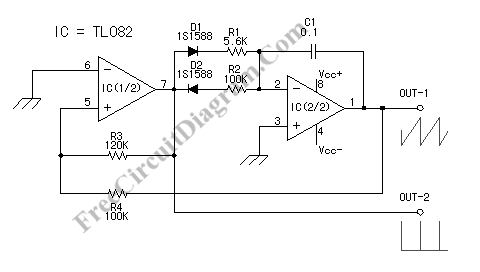## Colpitts Crystal Oscillator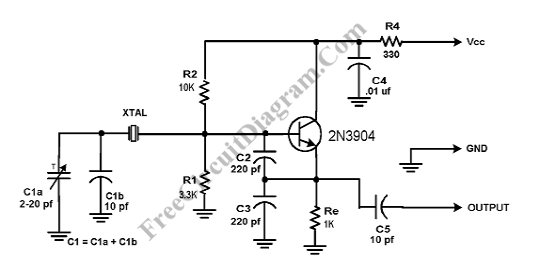Crystal Colpitts oscillator can be implemented using a transistor and a parallel mode crystal. Here is the schematic diagram of  the circuit: In this circuit, the crystal acts as an inductance. A large value capacitive divider is used between gate, source, and ground, and a small series capacitor is placed in the crystal circuit. You should choose the components values […]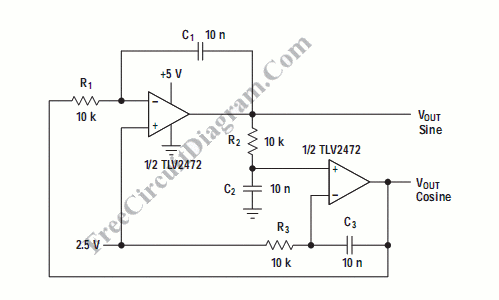Quadrature oscillator generate two sine-wave signal, but one signal is shifted 90 degree from the other signal. One output that lead the other called cosine signal, and the other is called sine. Here is the schematic diagram of the circuit: This circuit is another type of pahase shift oscillator, with three phase shifter sections. Each section is configured to produce […]

## Variable Frequency Sinewave Generator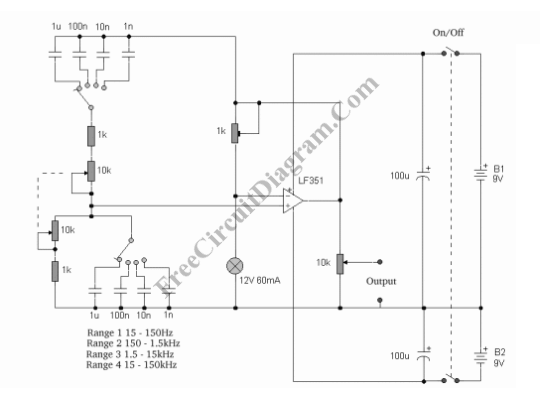This sine wave generator is adjustable between 15 Hz to 150 kHz. The circuit is basically a Wien-bridge oscillator, with multiple capacitor selection. Here is the schematic diagram: A stereo 10k potentiometer is used to control the frequency. The dashed line connecting these two potentiometers show that both potentiometer has single axis.

## High Frequency Waveform Generator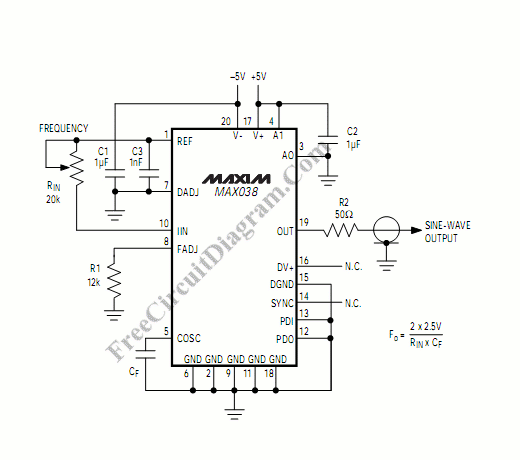Waveform generator is very useful in electronic experiment and design. This circuit generate sine wave oscillation, but actually we can modify the circuit to generate triangle or square wave function. Lets see its schematic diagram first, here it is: The core of this waveform generator is MAX038. This integrated circuit chip gives complete function to build a waveform generator/function generator.  […]

## 555 IC Oscillator with 50% Duty Cycle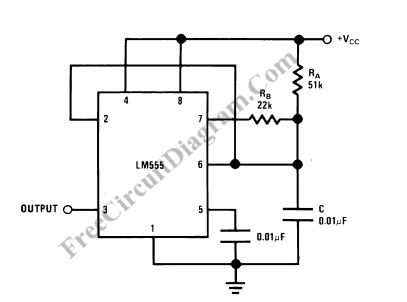A square wave oscillator using 555 IC can be configured to give symmetric oscillation (50% duty cycle). Other circuit uses diodes to split the charging and the discharging paths through different resistors, but here we need no such diodes. Here is the schematic diagram: The time period for the output high is the same as regular configuration, t1 = 0.693 […]

## Sawtooth Wave Oscillator Using 555 IC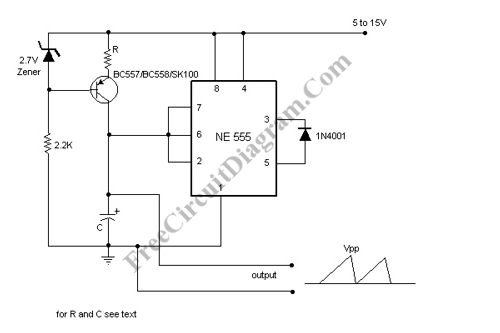Sawtooth wave oscillator  circuit can be implemented using several methods, here we present one design that employs 555 IC.  Here is the schematic diagram of the circuit: The core of the circuit uses  general 555 oscillator configuration, but instead of using a fixed resistor to limit the current for charging the capacitor, we use a constant current source. This change […]